# zero divide error on m1 chart !!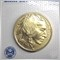1397

Hi can anyone fix the zero error divide ( the error is only when the indicator is put on m1 chart)

Thanks

:)

Files:
ForexMTN.mq4 3 kb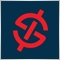54824

Please post the line numbers behind the zero divide error...1397

ForexMTN EURUSD,M1: zero divide in 'ForexMTN.mq4' (62,51)54824

This calculation of Minr seems to result in a zero

Minr = 0.2 * (Temp / 5.0);

And this causes the zero divide error in the following two lines

AboveBuff[i] = 3.0 * (High[i]  - Main) / Minr;

BelowBuff[i] = 3.0 * (Low[i]   - Main) / Minr;1397

thanks i fixed it :

i did

Temp = 0.000001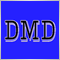4816

Another solution is :

```Minr = 0.2*(Temp*(MathPow(5,-1));
AboveBuff[i] = 3.0 * (High[i]  - Main) * (MathPow(Minr,-1));
BelowBuff[i] = 3.0 * (Low[i]   - Main) * (MathPow(Minr,-1));```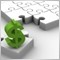8883

Always good to check any divisor in MQL that could give you a zero error. I always use a simple function:

```double dblNotZero(double aValue)
{
if (aValue==0) return(0.00001);
else return(aValue);
}```

Then pre-check any division that may produce a zero:

`X = Y / dblNotZero(Z);`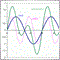37281

Stuart Browne:

Always good to check any divisor in MQL that could give you a zero error. I always use a simple function:

Then pre-check any division that may produce a zero: